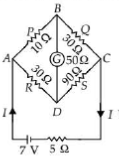KVL
Question

# The resistances of the four arms P, Q, Rand Sin a Wheatstone's bridge are 10 ohm, 30 ohm, 30 ohm and 90 ohm, respectively. The e.m.f. and internal resistance of the cell are 7 volt and 5 ohm respectively. 1f the galvanometer resistance is 50 ohm, the current drawn from the cell will be

Moderate
Solution

## The situation is as shown in the figure.For a balanced Wheatstone's bridgeIt is a balanced Wheatstone's bridge. Hence no current flows in the galvanometer arm. Hence, resistance 50 Q becomes ineffective.$\therefore$The equivalent resistance of the circuit isCurrent drawn from the cell is$I=\frac{7V}{35\Omega }=\frac{1}{5}A=0.2A$

Get Instant Solutions